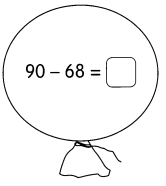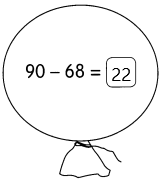Challenging Practice

The Hundred Train is here! On its cars are two numbers that add to 100.

Example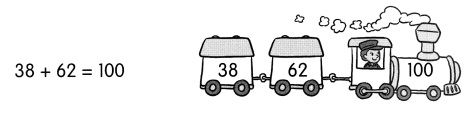There are many pairs of numbers that make 100. Write a pair of numbers on each train.

Question 1.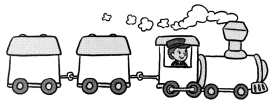45+55 = 100.

Explanation:
The pair of numbers that make 100 is 45 and 55.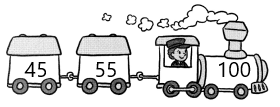Question 2.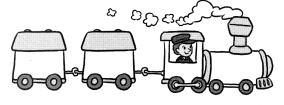35+65 = 100.

Explanation:
The pair of numbers that make 100 is 35 and 65.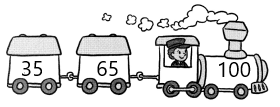Problem Solving

Rosa buys stickers each day. She buys 1 sticker on the 1st day, 2 stickers on the 2nd day, 3 stickers on the 3rd day and 4 stickers on the 4th day. Rosa follows this pattern for 10 days. How many stickers does she have in all?
The total number of stickers does she have in all is 55 stickers.

Explanation:
Given that Rosa buys stickers each day. She bought 1 sticker on the 1st day, 2 stickers on the 2nd day, 3 stickers on the 3rd day, and 4 stickers on the 4th day and the pattern follows for 10 days. So the number of stickers will be 1+2+3+4+5+6+7+8+9+10 which is 55 stickers.

Chapter Review/Test

Concepts and Skills

Question 1.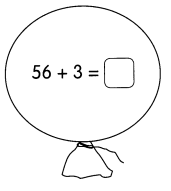56+3 = 59.

Explanation:
The sum of 56+3 is 59.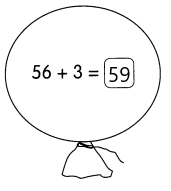Question 2.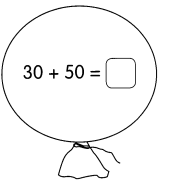30+50 = 80.

Explanation:
The sum of 30+50 is 80.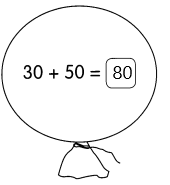Question 3.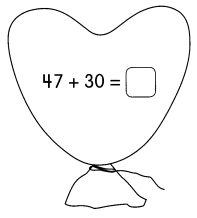47+30 = 77.

Explanation:
The sum of 47+30 is 77.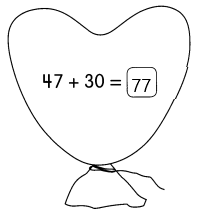Question 4.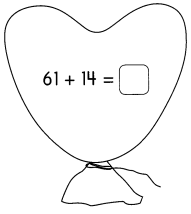61+14 = 75.

Explanation:
The sum of 61+14 is 75.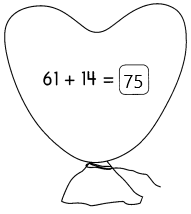Question 5.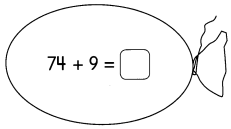74+9 = 83.

Explanation:
The sum of 74 and 9 is 83.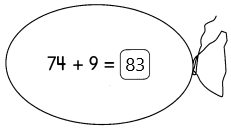Question 6.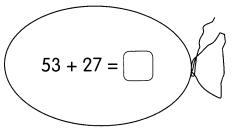53+27 = 80.

Explanation:
The sum of 53 and 27 is 80.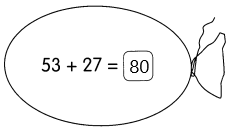Subtract.

Question 7.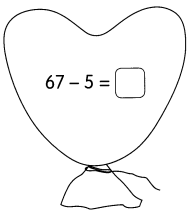67-5 = 62.

Explanation:
The subtraction of 67 and 5 is 62.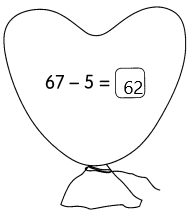Question 8.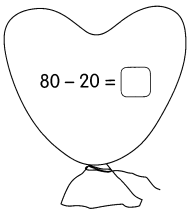80-20 = 60.

Explanation:
The subtraction of 80 and 20 is 60.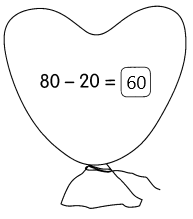Question 9.93 – 20 = 73.

Explanation:
The subtraction of 93 and 20 is 73.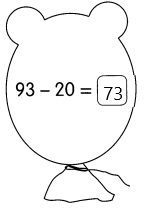Question 10.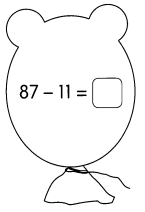87-11 = 76.

Explanation:
The subtraction of 87 and 11 is 76.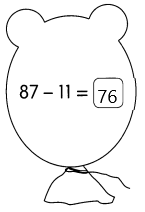Question 11.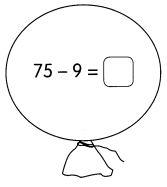75-9 = 66.

Explanation:
The subtraction of 75 and 9 is 66.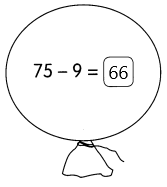Question 12.